# 【分形之美 03】彩色分形怎么画？分形上色与平滑插值讲解 来自深夜小编 CG文章_CG资源

## 分形的上色~

*文章授权转自微信公众号「包小猩CG杂货店」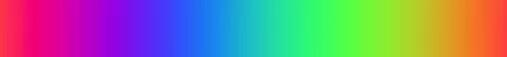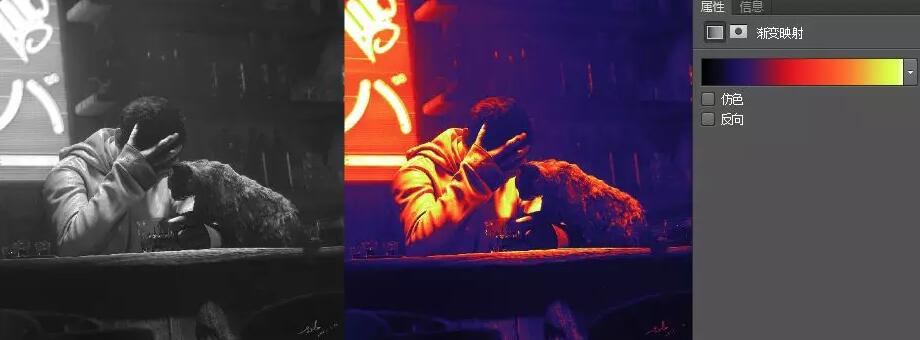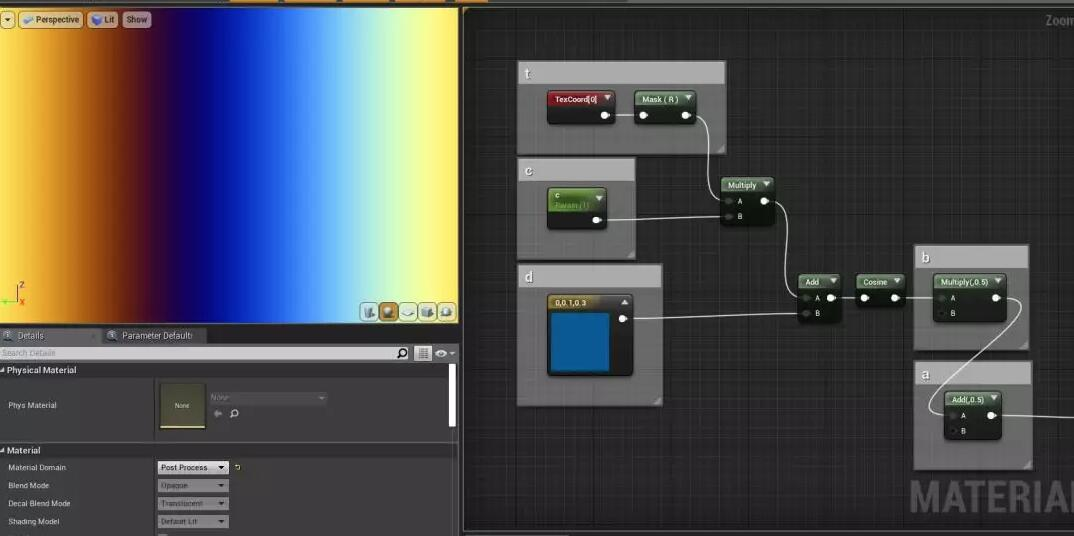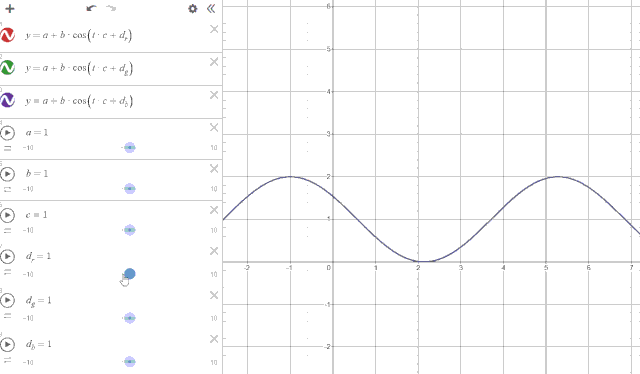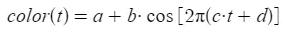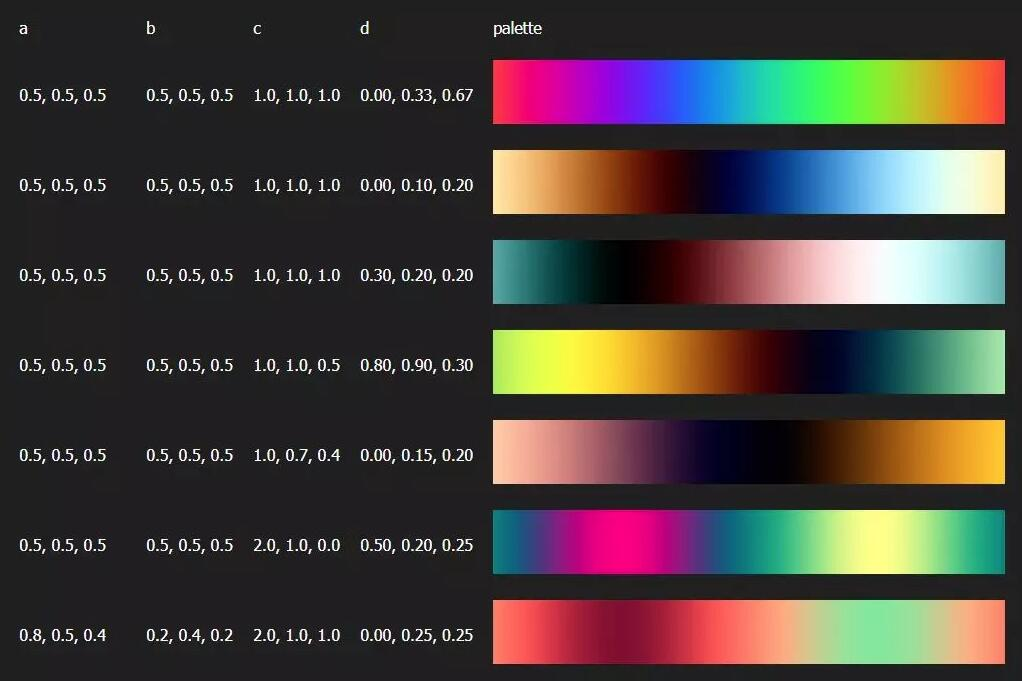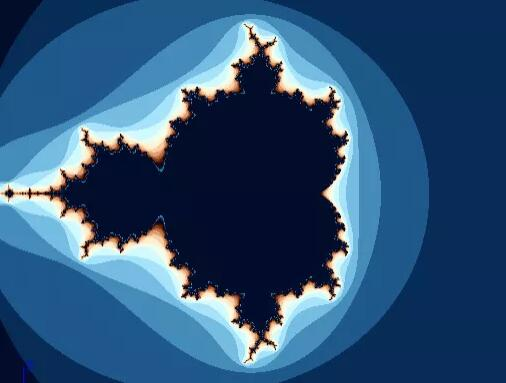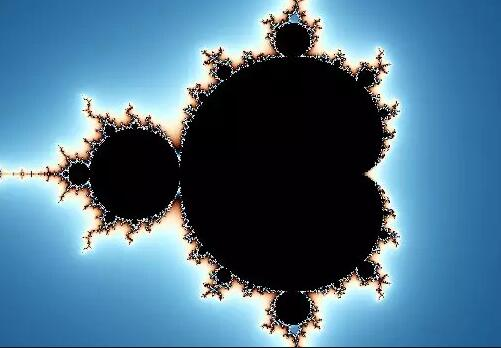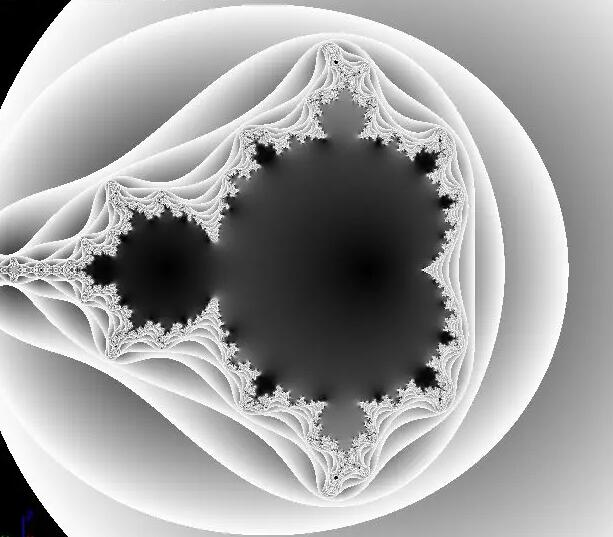z ：复变函数迭代的主体 ，起始值为 0，随后不断迭代

d ：分形函数的维度数 ，是一个 固定值 ，比如上面的图像维度为 2，d=2

c ：是一个复数 ，参与每次迭代，为 固定值

B ：最大值 ，如果某个点的z在迭代之后超过这个最大值，则迭代结束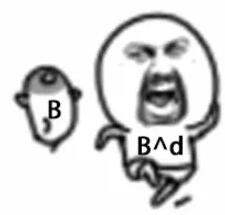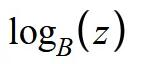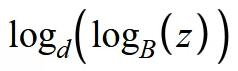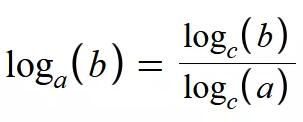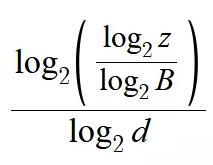（如果你要问为什么要这么麻烦去搞什么对数函数，不直接把 z 减个啥数再除一下就压缩回去了嘛，我只能说你这样是转不成线性变换的，为什么一定要纠结线性变换，因为最后每个环之间都要首尾衔接！！！不是线性变换衔接后的结果会非常不平滑，请自动脑补 5 个 s 形首尾相接的结果。）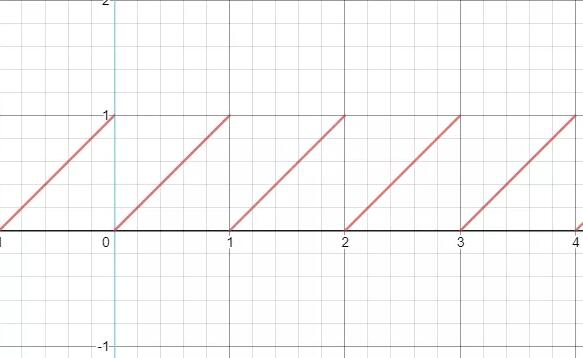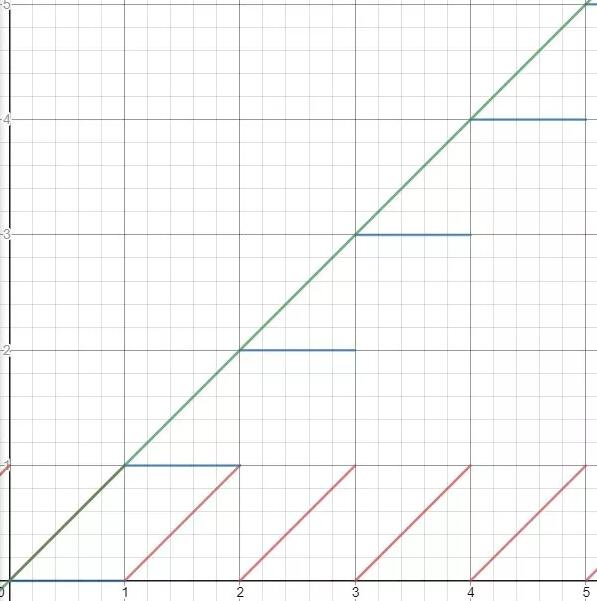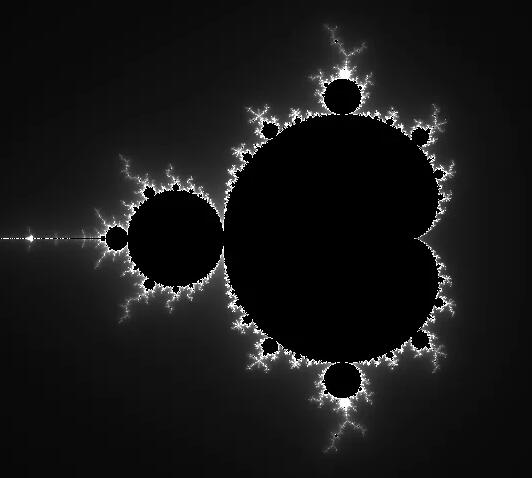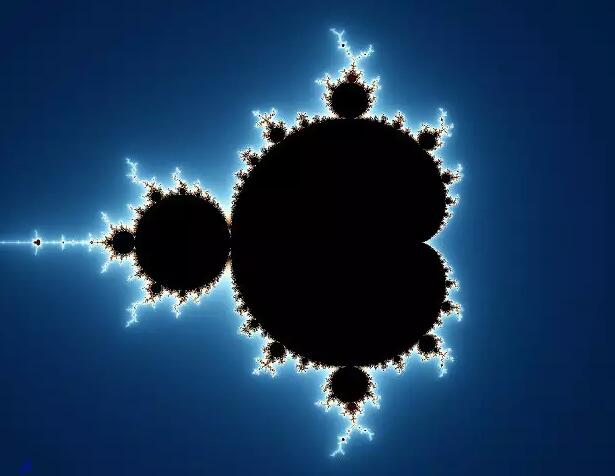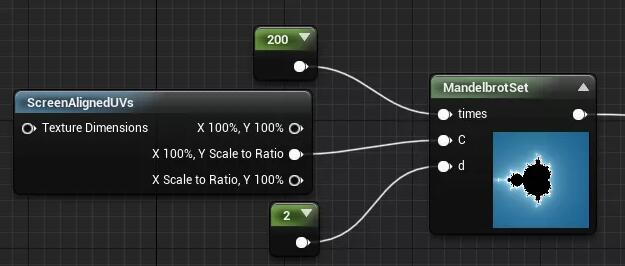// 统一定义一些必要的变量

float  xnew , ynew , n=0 , B = 60 , sit ;

float3 tmp;

float2 znew, z = (0,0);

// 将屏幕uv空间缩放至原来的两倍并将0点挪到屏幕中心

C = C * 4 - 2;

// 复变函数循环开始

for(int i = 0; i<times; i++)

{

z = float2(

cos( atan(z.y/z.x)*d)*pow(length(z),d),

sin( atan(z.y/z.x)*d)*pow(length(z),d)

)+C;

if(dot(z,z)>B*B)break;

// 循环次数加一（每个层级不一样）

n += 1;

}

// 将z的区间映射到0-1，然后和循环次数相减得到平滑的过渡

sit =n  - log2(log2(length(z))/(log2(B)))/log2(d);

//将映射结果运用颜色映射

tmp = 0.5 + 0.5*cos( 3.0 + sit*0.075*d + float3(0.0,0.6,1.0));

return tmp;